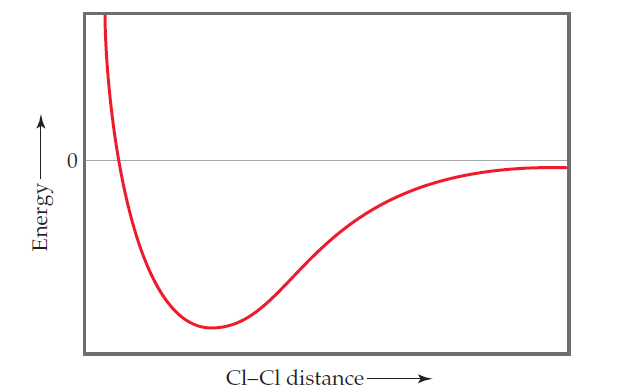×
Get Full Access to Chemistry: The Central Science - 12 Edition - Chapter 9 - Problem 5e
Get Full Access to Chemistry: The Central Science - 12 Edition - Chapter 9 - Problem 5e

×

# Visualizing ConceptsThe following plot shows the potentialISBN: 9780321696724 27

## Solution for problem 5E Chapter 9

Chemistry: The Central Science | 12th Edition

• Textbook Solutions
• 2901 Step-by-step solutions solved by professors and subject experts
• Get 24/7 help from StudySoup virtual teaching assistantsChemistry: The Central Science | 12th Edition

4 5 1 388 Reviews
29
3
Problem 5E

The following plot shows the potential energy of two Cl atoms as a function of the distance between them. (a) To what does an energy of zero correspond in this diagram? (b) According to the valence-bond model, why does the energy decrease as the Cl atoms move from a large separation to a smaller one? (c)What is the significance of the Cl¬Cl distance at the minimum point in the plot? (d) Why does the energy rise at Cl¬Cl distances less than that at the minimum point in the plot? (e) How can you estimate the bond strength of the Cl¬Cl bond from the plot? [Section 9.4]Step-by-Step Solution:
Step 1 of 3

Step 2 of 3

Step 3 of 3

##### ISBN: 9780321696724

The answer to “?The following plot shows the potential energy of two Cl atoms as a function of the distance between them. (a) To what does an energy of zero correspond in this diagram? (b) According to the valence-bond model, why does the energy decrease as the Cl atoms move from a large separation to a smaller one? (c)What is the significance of the Cl¬Cl distance at the minimum point in the plot? (d) Why does the energy rise at Cl¬Cl distances less than that at the minimum point in the plot? (e) How can you estimate the bond strength of the Cl¬Cl bond from the plot? [Section 9.4]” is broken down into a number of easy to follow steps, and 106 words. This full solution covers the following key subjects: plot, Energy, Bond, atoms, minimum. This expansive textbook survival guide covers 49 chapters, and 5471 solutions. This textbook survival guide was created for the textbook: Chemistry: The Central Science, edition: 12. Chemistry: The Central Science was written by and is associated to the ISBN: 9780321696724. Since the solution to 5E from 9 chapter was answered, more than 392 students have viewed the full step-by-step answer. The full step-by-step solution to problem: 5E from chapter: 9 was answered by , our top Chemistry solution expert on 04/03/17, 07:58AM.

Unlock Textbook Solution